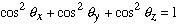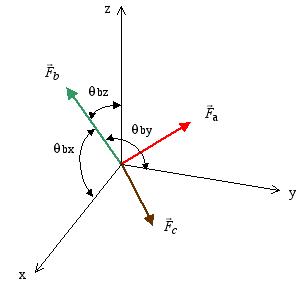Example 5: For the system of three non-coplanar concurrent forces shown, determine the x,y, z components and magnitude of the resultant force vector.

For clarity only the angles for force Fb are shown in the figure below. For the other two force vectors the angles are measured in a similar way from positive x,y,z axes. Keep in mind that only two out of three direction angles are specified as the third angle can be found from the relationNOTE: In this particular example problem, changing the values of the force magnitudes will result in the resultant having an imaginary component. This is an error in the LiveMath plugin for Windows 9x. The real component of the resultant is correct, so the imaginary component may be ignored.